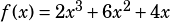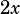test number 4 section 4 question number 25 indicates
Choice B is correct.
they the college board explains:
In f(x), factoring out the greatest common factor, 2x, yields
f(x) = 2x (x2 + 3x + 2).

however I do not find 2x to be a factor of X^3 +6X +4 because the 4 has no X associated with it. I did not get the answer as b either but c. Could you explain?

Thx John

I’ve got a full explanation of that question here (you can see all the official test questions I’ve explained here).

I think the problem you’re having is related to the initial factoring. You’re originally told that. To factor aout of that, you need to pull it out of each term (i.e, out of each part of the expression separated by a plus or minus sign). Here’s what your work might look like:Does that help?